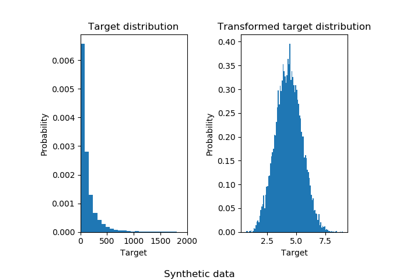# sklearn.preprocessing.quantile_transform¶

sklearn.preprocessing.quantile_transform(X, axis=0, n_quantiles=1000, output_distribution='uniform', ignore_implicit_zeros=False, subsample=100000, random_state=None, copy=False)[source]

Transform features using quantiles information.

This method transforms the features to follow a uniform or a normal distribution. Therefore, for a given feature, this transformation tends to spread out the most frequent values. It also reduces the impact of (marginal) outliers: this is therefore a robust preprocessing scheme.

The transformation is applied on each feature independently. The cumulative distribution function of a feature is used to project the original values. Features values of new/unseen data that fall below or above the fitted range will be mapped to the bounds of the output distribution. Note that this transform is non-linear. It may distort linear correlations between variables measured at the same scale but renders variables measured at different scales more directly comparable.

Read more in the User Guide.

Parameters: X : array-like, sparse matrix The data to transform. axis : int, (default=0) Axis used to compute the means and standard deviations along. If 0, transform each feature, otherwise (if 1) transform each sample. n_quantiles : int, optional (default=1000) Number of quantiles to be computed. It corresponds to the number of landmarks used to discretize the cumulative distribution function. output_distribution : str, optional (default=’uniform’) Marginal distribution for the transformed data. The choices are ‘uniform’ (default) or ‘normal’. ignore_implicit_zeros : bool, optional (default=False) Only applies to sparse matrices. If True, the sparse entries of the matrix are discarded to compute the quantile statistics. If False, these entries are treated as zeros. subsample : int, optional (default=1e5) Maximum number of samples used to estimate the quantiles for computational efficiency. Note that the subsampling procedure may differ for value-identical sparse and dense matrices. random_state : int, RandomState instance or None, optional (default=None) If int, random_state is the seed used by the random number generator; If RandomState instance, random_state is the random number generator; If None, the random number generator is the RandomState instance used by np.random. Note that this is used by subsampling and smoothing noise. copy : boolean, optional, (default=True) Set to False to perform inplace transformation and avoid a copy (if the input is already a numpy array). quantiles_ : ndarray, shape (n_quantiles, n_features) The values corresponding the quantiles of reference. references_ : ndarray, shape(n_quantiles, ) Quantiles of references.

QuantileTransformer
Performs quantile-based scaling using the Transformer API (e.g. as part of a preprocessing sklearn.pipeline.Pipeline).
power_transform
Maps data to a normal distribution using a power transformation.
scale
Performs standardization that is faster, but less robust to outliers.
robust_scale
Performs robust standardization that removes the influence of outliers but does not put outliers and inliers on the same scale.

Notes

NaNs are treated as missing values: disregarded in fit, and maintained in transform.

For a comparison of the different scalers, transformers, and normalizers, see examples/preprocessing/plot_all_scaling.py.

Examples

>>> import numpy as np
>>> from sklearn.preprocessing import quantile_transform
>>> rng = np.random.RandomState(0)
>>> X = np.sort(rng.normal(loc=0.5, scale=0.25, size=(25, 1)), axis=0)
>>> quantile_transform(X, n_quantiles=10, random_state=0)
...
array([...])


## Examples using sklearn.preprocessing.quantile_transform¶Effect of transforming the targets in regression model